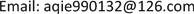﻿ 时滞随机控制系统解的估计 Estimation of Solutions for Stochastic Control Systems with Time Delays

Vol. 08  No. 10 ( 2019 ), Article ID: 32467 , 5 pages
10.12677/AAM.2019.810187

Estimation of Solutions for Stochastic Control Systems with Time Delays

Jie Xu, Xiaomei Cui

College of Science, Jilin Institute of Chemical Technology, Jilin JilinReceived: Sep. 24th, 2019; accepted: Oct. 4th, 2019; published: Oct. 11th, 2019ABSTRACT

Estimation of solutions for stochastic time delay systems is an important basis for the problem of optimal control systems with time delay. In this paper, we estimate the solution of the state equation of time delay control systems for the general case by using Cauchy-Schwarz and Gronwall inequalities. We use two methods to prove our conclusions, and lay a theoretical foundation for further study of time-delay control problems. And we hope to lay a theoretical foundation for further research on time-delay control.

Keywords:Time Delay System, Estimation, Cauchy-Schwarz Inequality, Gronwall Inequality1. 引言

2. 符号介绍

$\left(\Omega ,F,P\right)$ 是完备的概率空间， ${\left\{{F}_{t}\right\}}_{t\ge 0}$ 是由标准布朗运动 $W\left(t\right)$ 生成的域流。 ${\delta }_{1}$${\delta }_{2}$ 和T是已知常数。 ${L}_{F}^{2}\left(0,T;{R}^{m}\right)$ 表示 ${R}^{m}$${F}_{t}$ 适应过程 $\left\{X\left(t\right),0\le t\le T\right\}$ 的空间，其中 $E\left[{\int }_{s}^{r}{|X\left(t\right)|}^{2}\text{d}t\right]<\infty$$\zeta :\left[-{\delta }_{1},0\right]\to {R}^{n}$ 是连续函数。

$\left\{\begin{array}{l}\text{d}x\left(t\right)=b\left(t,x\left(t\right),x\left(t-{\delta }_{1}\right),u\left(t\right),u\left(t-{\delta }_{2}\right)\right)\text{d}t+\sigma \left(t,x\left(t\right),x\left(t-{\delta }_{1}\right),u\left(t\right),u\left(t-{\delta }_{2}\right)\right)\text{d}W\left(t\right),\\ x\left(t\right)=\zeta \left(t\right),\text{\hspace{0.17em}}t\in \left[-{\delta }_{1},0\right]\end{array}$ (1)

$J\left(u\left(\cdot \right)\right)=E\left\{{\int }_{0}^{T}\left\{L\left(t\right),x\left(t\right),u\left(t\right)\right\}\text{d}t+\Phi \left(x\left(T\right)\right)\right\}$ (2)

(A1) 存在常数 $C>0$ 使得对任意 ${x}_{1}\left(t\right),{x}_{2}\left(t\right),{x}_{1}\left(t-\delta \right),{x}_{2}\left(t-\delta \right),{u}_{1}\left(t\right),{u}_{2}\left(t\right),{u}_{1}\left(t-\delta \right),{u}_{2}\left(t-\delta \right)$ 下面不等式成立：

$\begin{array}{l}{|\rho \left(t,{x}_{1}\left(t\right),{x}_{1}\left(t-{\delta }_{1}\right),{u}_{1}\left(t\right),{u}_{1}\left(t-{\delta }_{2}\right)\right)-b\left(t,{x}_{2}\left(t\right),{x}_{2}\left(t-{\delta }_{1}\right),{u}_{2}\left(t\right),{u}_{2}\left(t-{\delta }_{2}\right)\right)|}^{2}\\ \le C\left[{|{x}_{1}\left(t\right)-{x}_{2}\left(t\right)|}^{2}+{|{x}_{1}\left(t-{\delta }_{2}\right)-{x}_{2}\left(t-{\delta }_{2}\right)|}^{2}+{|{u}_{1}\left(t\right)-{u}_{2}\left(t\right)|}^{2}+{|{u}_{1}\left(t-{\delta }_{2}\right)-{u}_{2}\left(t-{\delta }_{2}\right)|}^{2}\right]\end{array}$

$\Gamma$ 是非空的凸控制域， $U\left[0,T\right]\subset \Gamma$ 表示可容控制集合， $u\left(t\right)$ 定义为

$u\left(t\right)=\left\{\begin{array}{l}0,\text{ }t\in \left[-{\delta }_{2},0\right]\\ u\left(t\right)\in {L}_{F}^{2}\left(0,T;{R}^{m}\right)且u\left(t\right)\in U,\text{ }t\in \left[0,T\right]\end{array}$

3. 主要内容

$\begin{array}{l}E\left[\underset{0\le t\le T}{\mathrm{sup}}{|{x}_{1}\left(t\right)-{x}_{2}\left(t\right)|}^{2}\right]\\ \le C\left\{E{\int }_{0}^{T}{|b\left(t,{x}_{1}\left(t\right),{x}_{1}\left(t-{\delta }_{1}\right),{u}_{1}\left(t\right),{u}_{1}\left(t-{\delta }_{2}\right)\right)-b\left(t,{x}_{2}\left(t\right),{x}_{2}\left(t-{\delta }_{1}\right),{u}_{2}\left(t\right),{u}_{2}\left(t-{\delta }_{2}\right)\right)|}^{2}\text{d}t\\ \text{\hspace{0.17em}}\text{\hspace{0.17em}}\text{ }+E{\int }_{0}^{T}{|\sigma \left(t,{x}_{1}\left(t\right),{x}_{1}\left(t-{\delta }_{1}\right),{u}_{1}\left(t\right),{u}_{1}\left(t-{\delta }_{2}\right)\right)-\sigma \left(t,{x}_{2}\left(t\right),{x}_{2}\left(t-{\delta }_{1}\right),{u}_{2}\left(t\right),{u}_{2}\left(t-{\delta }_{2}\right)\right)|}^{2}\text{d}t\right\}\end{array}$ (3)

$\begin{array}{l}E{\int }_{0}^{T}{|b\left(t,{x}_{1}\left(t\right),{x}_{1}\left(t-{\delta }_{1}\right),{u}_{1}\left(t\right),{u}_{1}\left(t-{\delta }_{2}\right)\right)-b\left(t,{x}_{2}\left(t\right),{x}_{2}\left(t-{\delta }_{1}\right),{u}_{2}\left(t\right),{u}_{2}\left(t-{\delta }_{2}\right)\right)|}^{2}\text{d}t\\ \le E{\int }_{0}^{T}{|b\left(t,{x}_{1}\left(t\right),{x}_{1}\left(t-{\delta }_{1}\right),{u}_{1}\left(t\right),{u}_{2}\left(t-{\delta }_{2}\right)\right)-b\left(t,{x}_{1}\left(t\right),{x}_{1}\left(t-{\delta }_{1}\right),{u}_{2}\left(t\right),{u}_{2}\left(t-{\delta }_{2}\right)\right)|}^{2}\text{d}t\\ \text{\hspace{0.17em}}\text{\hspace{0.17em}}+E{\int }_{0}^{T}{|b\left(t,{x}_{1}\left(t\right),{x}_{1}\left(t-{\delta }_{1}\right),{u}_{2}\left(t\right),{u}_{2}\left(t-{\delta }_{2}\right)\right)-b\left(t,{x}_{1}\left(t\right),{x}_{2}\left(t-{\delta }_{1}\right),{u}_{2}\left(t\right),{u}_{2}\left(t-{\delta }_{2}\right)\right)|}^{2}\text{d}t\\ \text{\hspace{0.17em}}\text{\hspace{0.17em}}+E{\int }_{0}^{T}{|b\left(t,{x}_{1}\left(t\right),{x}_{1}\left(t-{\delta }_{1}\right),{u}_{1}\left(t\right),{u}_{1}\left(t-{\delta }_{2}\right)\right)-b\left(t,{x}_{1}\left(t\right),{x}_{1}\left(t-{\delta }_{1}\right),{u}_{1}\left(t\right),{u}_{2}\left(t-{\delta }_{2}\right)\right)|}^{2}\text{d}t\\ \text{\hspace{0.17em}}\text{\hspace{0.17em}}+E{\int }_{0}^{T}{|b\left(t,{x}_{1}\left(t\right),{x}_{2}\left(t-{\delta }_{1}\right),{u}_{2}\left(t\right),{u}_{2}\left(t-{\delta }_{2}\right)\right)-b\left(t,{x}_{2}\left(t\right),{x}_{2}\left(t-{\delta }_{1}\right),{u}_{2}\left(t\right),{u}_{2}\left(t-{\delta }_{2}\right)\right)|}^{2}\text{d}t\end{array}$

$\begin{array}{l}\le E{\int }_{0}^{T}{|{u}_{1}\left(t\right)-{u}_{2}\left(t\right)|}^{2}\text{d}t+E{\int }_{0}^{T}{|{u}_{1}\left(t-{\delta }_{2}\right)-{u}_{2}\left(t-{\delta }_{2}\right)|}^{2}\text{d}t\\ \text{\hspace{0.17em}}\text{\hspace{0.17em}}+E{\int }_{0}^{T}{|{x}_{1}\left(t\right)-{x}_{2}\left(t\right)|}^{2}\text{d}t+E{\int }_{0}^{T}{|{x}_{1}\left(t-{\delta }_{1}\right)-{x}_{2}\left(t-{\delta }_{1}\right)|}^{2}\text{d}t\end{array}$ (4)

$\begin{array}{l}E{\int }_{0}^{T}{|{u}_{1}\left(t-{\delta }_{2}\right)-{u}_{2}\left(t-{\delta }_{2}\right)|}^{2}\text{d}t\\ =E{\int }_{-{\delta }_{2}}^{T-{\delta }_{2}}{|{u}_{1}\left(t\right)-{u}_{2}\left(t\right)|}^{2}\text{d}t\\ =E{\int }_{-{\delta }_{2}}^{0}{|{u}_{1}\left(t\right)-{u}_{2}\left(t\right)|}^{2}\text{d}t+E{\int }_{0}^{T-{\delta }_{2}}{|{u}_{1}\left(t\right)-{u}_{2}\left(t\right)|}^{2}\text{d}t\\ \le E{\int }_{0}^{T}{|{u}_{1}\left(t\right)-{u}_{2}\left(t\right)|}^{2}\text{d}t\\ =d{\left({u}_{1}\left(t\right),{u}_{2}\left(t\right)\right)}^{2}\end{array}$ (5)

$\begin{array}{l}E{\int }_{0}^{T}{|{x}_{1}\left(t-{\delta }_{2}\right)-{x}_{2}\left(t-{\delta }_{2}\right)|}^{2}\text{d}t\\ =E{\int }_{-{\delta }_{2}}^{T-{\delta }_{2}}{|{x}_{1}\left(t\right)-{x}_{2}\left(t\right)|}^{2}\text{d}t\\ =E{\int }_{-{\delta }_{2}}^{0}{|{x}_{1}\left(t\right)-{x}_{2}\left(t\right)|}^{2}\text{d}t+E{\int }_{0}^{T-{\delta }_{2}}{|{x}_{1}\left(t\right)-{x}_{2}\left(t\right)|}^{2}\text{d}t\\ \le E{\int }_{0}^{T}{|{x}_{1}\left(t\right)-{x}_{2}\left(t\right)|}^{2}\text{d}t\end{array}$ (6)

$\begin{array}{l}E{\int }_{0}^{T}{|b\left(t,{x}_{1}\left(t\right),{x}_{1}\left(t-{\delta }_{1}\right),{u}_{1}\left(t\right),{u}_{1}\left(t-{\delta }_{2}\right)\right)-b\left(t,{x}_{2}\left(t\right),{x}_{2}\left(t-{\delta }_{1}\right),{u}_{2}\left(t\right),{u}_{2}\left(t-{\delta }_{2}\right)\right)|}^{2}\text{d}t\\ \le E{\int }_{0}^{T}{|{u}_{1}\left(t\right)-{u}_{2}\left(t\right)|}^{2}\text{d}t+E{\int }_{0}^{T}{|{u}_{1}\left(t-{\delta }_{2}\right)-{u}_{2}\left(t-{\delta }_{2}\right)|}^{2}\text{d}t\\ \text{\hspace{0.17em}}\text{\hspace{0.17em}}\text{ }+E{\int }_{0}^{T}{|{x}_{1}\left(t\right)-{x}_{2}\left(t\right)|}^{2}\text{d}t+E{\int }_{0}^{T}{|{x}_{1}\left(t-{\delta }_{2}\right)-{x}_{2}\left(t-{\delta }_{2}\right)|}^{2}\text{d}t\\ \le d\left({u}_{1}\left(t\right),{u}_{2}\left(t\right)\right)+E{\int }_{0}^{T}{|{x}_{1}\left(t\right)-{x}_{2}\left(t\right)|}^{2}\text{d}t\end{array}$ (7)

$\begin{array}{l}E{\int }_{0}^{T}{|\sigma \left(t,{x}_{1}\left(t\right),{x}_{1}\left(t-{\delta }_{1}\right),{u}_{1}\left(t\right),{u}_{1}\left(t-{\delta }_{2}\right)\right)-\sigma \left(t,{x}_{2}\left(t\right),{x}_{2}\left(t-{\delta }_{1}\right),{u}_{2}\left(t\right),{u}_{2}\left(t-{\delta }_{2}\right)\right)|}^{2}\text{d}t\\ \le E{\int }_{0}^{T}{|\sigma \left(t,{x}_{1}\left(t\right),{x}_{1}\left(t-{\delta }_{1}\right),{u}_{1}\left(t\right),{u}_{2}\left(t-{\delta }_{2}\right)\right)-\sigma \left(t,{x}_{1}\left(t\right),{x}_{1}\left(t-{\delta }_{1}\right),{u}_{2}\left(t\right),{u}_{2}\left(t-{\delta }_{2}\right)\right)|}^{2}\text{d}t\\ \text{\hspace{0.17em}}\text{\hspace{0.17em}}+E{\int }_{0}^{T}{|\sigma \left(t,{x}_{1}\left(t\right),{x}_{1}\left(t-{\delta }_{1}\right),{u}_{2}\left(t\right),{u}_{2}\left(t-{\delta }_{2}\right)\right)-\sigma \left(t,{x}_{1}\left(t\right),{x}_{2}\left(t-{\delta }_{1}\right),{u}_{2}\left(t\right),{u}_{2}\left(t-{\delta }_{2}\right)\right)|}^{2}\text{d}t\\ \text{\hspace{0.17em}}\text{\hspace{0.17em}}+E{\int }_{0}^{T}{|\sigma \left(t,{x}_{1}\left(t\right),{x}_{1}\left(t-{\delta }_{1}\right),{u}_{1}\left(t\right),{u}_{1}\left(t-{\delta }_{2}\right)\right)-\sigma \left(t,{x}_{1}\left(t\right),{x}_{1}\left(t-{\delta }_{1}\right),{u}_{1}\left(t\right),{u}_{2}\left(t-{\delta }_{2}\right)\right)|}^{2}\text{d}t\\ \text{\hspace{0.17em}}\text{\hspace{0.17em}}+E{\int }_{0}^{T}{|\sigma \left(t,{x}_{1}\left(t\right),{x}_{2}\left(t-{\delta }_{1}\right),{u}_{2}\left(t\right),{u}_{2}\left(t-{\delta }_{2}\right)\right)-\sigma \left(t,{x}_{2}\left(t\right),{x}_{2}\left(t-{\delta }_{1}\right),{u}_{2}\left(t\right),{u}_{2}\left(t-{\delta }_{2}\right)\right)|}^{2}\text{d}t\end{array}$

$\begin{array}{l}\le E{\int }_{0}^{T}{|{u}_{1}\left(t\right)-{u}_{2}\left(t\right)|}^{2}\text{d}t+E{\int }_{0}^{T}{|{u}_{1}\left(t-{\delta }_{2}\right)-{u}_{2}\left(t-{\delta }_{2}\right)|}^{2}\text{d}t\\ \text{\hspace{0.17em}}\text{\hspace{0.17em}}+E{\int }_{0}^{T}{|{x}_{1}\left(t\right)-{x}_{2}\left(t\right)|}^{2}\text{d}t+E{\int }_{0}^{T}{|{x}_{1}\left(t-{\delta }_{2}\right)-{x}_{2}\left(t-{\delta }_{2}\right)|}^{2}\text{d}t\\ \le d\left({u}_{1}\left(t\right),{u}_{2}\left(t\right)\right)+E{\int }_{0}^{T}{|{x}_{1}\left(t\right)-{x}_{2}\left(t\right)|}^{2}\text{d}t\end{array}$ (8)

$\begin{array}{l}E\left[\underset{0\le t\le T}{\mathrm{sup}}{|{x}_{1}\left(t\right)-{x}_{2}\left(t\right)|}^{2}\right]\\ \le C\left\{E{\int }_{0}^{T}{|b\left(t,{x}_{1}\left(t\right),{x}_{1}\left(t-{\delta }_{1}\right),{u}_{1}\left(t\right),{u}_{1}\left(t-{\delta }_{2}\right)\right)-b\left(t,{x}_{2}\left(t\right),{x}_{2}\left(t-{\delta }_{1}\right),{u}_{2}\left(t\right),{u}_{2}\left(t-{\delta }_{2}\right)\right)|}^{2}\text{d}t\\ \text{\hspace{0.17em}}\text{\hspace{0.17em}}+E{\int }_{0}^{T}{|\sigma \left(t,{x}_{1}\left(t\right),{x}_{1}\left(t-{\delta }_{1}\right),{u}_{1}\left(t\right),{u}_{1}\left(t-{\delta }_{2}\right)\right)-\sigma \left(t,{x}_{2}\left(t\right),{x}_{2}\left(t-{\delta }_{1}\right),{u}_{2}\left(t\right),{u}_{2}\left(t-{\delta }_{2}\right)\right)|}^{2}\text{d}t\right\}\\ \le C\left\{d{\left({u}_{1}\left(t\right),{u}_{2}\left(t\right)\right)}^{2}+{\int }_{0}^{T}E\left[\underset{0\le t\le \theta }{\mathrm{sup}}{|{x}_{1}\left(t\right)-{x}_{2}\left(t\right)|}^{2}\right]\text{d}\theta \right\}\end{array}$

$\begin{array}{l}{|b\left(t,{x}_{1}\left(t\right),{x}_{1}\left(t-{\delta }_{1}\right),{u}_{1}\left(t\right),{u}_{1}\left(t-{\delta }_{2}\right)\right)-b\left(t,{x}_{2}\left(t\right),{x}_{2}\left(t-{\delta }_{1}\right),{u}_{2}\left(t\right),{u}_{2}\left(t-{\delta }_{2}\right)\right)|}^{2}\\ \le C\left[{|{u}_{1}\left(t\right)-{u}_{2}\left(t\right)|}^{2}+{|{u}_{1}\left(t-{\delta }_{2}\right)-{u}_{2}\left(t-{\delta }_{2}\right)|}^{2}\right]\end{array}$

$\begin{array}{l}E{\int }_{0}^{T}{|b\left(t,{x}_{1}\left(t\right),{x}_{1}\left(t-{\delta }_{1}\right),{u}_{1}\left(t\right),{u}_{1}\left(t-{\delta }_{2}\right)\right)-b\left(t,{x}_{1}\left(t\right),{x}_{1}\left(t-{\delta }_{1}\right),{u}_{2}\left(t\right),{u}_{2}\left(t-{\delta }_{2}\right)\right)|}^{2}\text{d}t\\ \le CE{\int }_{0}^{T}{|{u}_{1}\left(t\right)-{u}_{2}\left(t\right)|}^{2}\text{d}t+CE{\int }_{0}^{T}{|{u}_{1}\left(t-{\delta }_{2}\right)-{u}_{2}\left(t-{\delta }_{2}\right)|}^{2}\text{d}t\\ =CE{\int }_{0}^{T}{|{u}_{1}\left(t\right)-{u}_{2}\left(t\right)|}^{2}\text{d}t+CE{\int }_{-{\delta }_{2}}^{T-{\delta }_{2}}{|{u}_{1}\left(t\right)-{u}_{2}\left(t\right)|}^{2}\text{d}t\\ =CE{\int }_{0}^{T}{|{u}_{1}\left(t\right)-{u}_{2}\left(t\right)|}^{2}\text{d}t+CE{\int }_{-{\delta }_{2}}^{0}{|{u}_{1}\left(t\right)-{u}_{2}\left(t\right)|}^{2}\text{d}t+CE{\int }_{0}^{T-{\delta }_{2}}{|{u}_{1}\left(t\right)-{u}_{2}\left(t\right)|}^{2}\text{d}t\\ \le CE{\int }_{0}^{T}{|{u}_{1}\left(t\right)-{u}_{2}\left(t\right)|}^{2}\text{d}t+CE{\int }_{0}^{T}{|{u}_{1}\left(t\right)-{u}_{2}\left(t\right)|}^{2}\text{d}t\\ \le Cd{\left({u}_{1}\left(t\right),{u}_{2}\left(t\right)\right)}^{2}\end{array}$

$\begin{array}{l}E{\int }_{0}^{T}{|\sigma \left(t,{x}_{1}\left(t\right),{x}_{1}\left(t-{\delta }_{1}\right),{u}_{1}\left(t\right),{u}_{1}\left(t-{\delta }_{2}\right)\right)-\sigma \left(t,{x}_{1}\left(t\right),{x}_{1}\left(t-{\delta }_{1}\right),{u}_{2}\left(t\right),{u}_{2}\left(t-{\delta }_{2}\right)\right)|}^{2}\text{d}t\\ \le Cd{\left({u}_{1}\left(t\right),{u}_{2}\left(t\right)\right)}^{2}\end{array}$

Estimation of Solutions for Stochastic Control Systems with Time Delays[J]. 应用数学进展, 2019, 08(10): 1597-1601. https://doi.org/10.12677/AAM.2019.810187

1. 1. Zhou, X.Y. (1998) Stochastic Near-Optimal Controls: Necessary and Sufficient Conditions for Near-Optimality. SIAM Journal on Control and Optimization, 36, 929-947.
https://doi.org/10.1137/s0363012996302664

2. 2. Zhang, F. (2017) Maximum Principle for Near-Optimality of Stochastic Delay Control Problem. Advances in Difference Equations, 98.
https://doi.org/10.1186/s13662-017-1155-9

3. 3. Wang, Y. and Wu, Z. (2017) Necessary and Sufficient Conditions for Near-Optimality of Stochastic Delay Systems. International Journal of Control, 91, 1730-1744.
https://doi.org/10.1080/00207179.2017.1327725

4. 4. 潘立平, Koklay. 广义时间最优控制问题的近似最优解[J]. 数学年刊: A辑, 1998(5): 601-612.

5. 5. 齐斌. 具有时滞的广义时间最优控制问题的近似最优解[J]. 东莞理工学院学报, 2007, 14(1): 22-25.

6. 6. 杨园华, 韩春艳, 刘晓华, 等. 有界随机测量时滞的网络控制系统的最优估计[J]. 控制理论与应用, 2014, 31(2): 181-187.

7. 7. 王青丽. 时滞随机系统的估计和控制[D]: [硕士学位论文]. 曲阜: 曲阜师范大学, 2011.

8. 8. 韩春艳. Markovian随机时滞系统的状态估计[D]: [博士学位论文]. 济南: 山东大学, 2010.